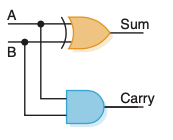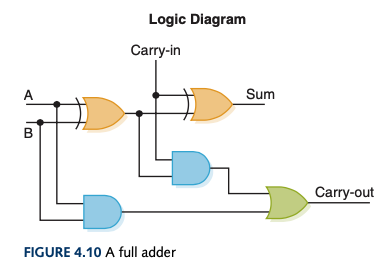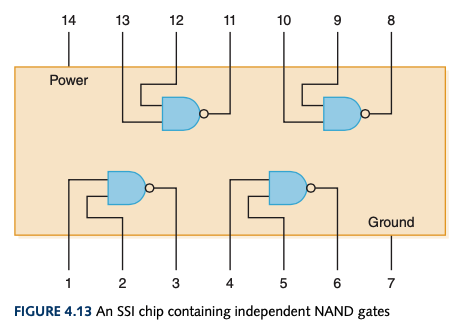# Circuit

• A bunch of logic gates combined
• Output of one gate --> input of another
• Flow of electricity is controlled by the interacting gates
• Equivalent when they produce the same output for identical input

## Combinational Circuits

• Circuit whose output is solely determined by its input values

## Sequential Circuits

• Circuit whose output is a function of its input values and the current state of the circuit

• Performs addition operation on binary values• Computes the sum of two bits and produces the appropriate carry bit
• An XOR and an AND gate
• Doesn't allow a possible carry coming in the calculation• Computes the sum of two bits, taking an input carry bit into account

## Multiplexers

• Input control signals determine which input is routed to its output
• Many input lines
• Often called a mux
• Values on $n$ input control lines determine which of $2^n$ other data lines are selected for output
• Demultiplexer (demux) is the opposite of a mux
• Single input --> one of $2^n$ outputs depending on values of $n$ control lines

## Circuits as Memory

• Can store information
• Sequential circuit
• Output serves as input
• Existing state of circuit is used to determine next state

### S-R Latch• Stores single bit
• Guarantees two outputs ($X$ and $Y$) are always complements of each other

## Integrated Circuits